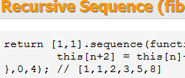## Array.Math 0.1.4Array.Math is a collection of calculation with arrays. You can do all kind of things with arrays. You can see them as data for statistic analysis, you can see them as vectors and matrices for linear algebra, or just use them for calculus like add, subtract and products or more advanced calculus with sequences.

The next steps are Vectors and Matrices. The aim is to be able to calculate eigenvalues and eigenvectors from a given Matrix. Singular Value Decomposition would be nice as well. To do this, JavaScript needs to be extended so it can handle Complex numbers, this can be found in Complex.js

To be sure all the methods work as expected, there is a Specs folder that contains some tests.

Author
Arian Stolwijk
Current version
0.1.4
GitHub
arian/Array.Math
15826
Category
Native
Tags
Report
GitHub Issues

### Dependencies

• _self_/_current_:
• Core/Array
• Core/Class
• Core/Number
• Vector
• Complex/Complex
• Array.Math

### Array:toNumbers

Transforms each value of the array to a number.

```['1', '13', '300'].toNumbers(); // [1, 13, 300]
```

### Array:sortNumbers

Sorts an array as numbers. The sort method usually transforms each value to a string and lexically compares the values. This will result that '10' will before '2'. This is a quick method to fix that.

```[99, 2, 4, 40, 10].sortNumbers(); [2, 4, 10, 40, 99]
```

### Array:sum

Sum all the entities of the array

```[2, 5, 1, 6].sum(); // 14
[2, 5, 2, 7, 7, 12, 8].sum([start], [length])
```

### Array:product

Get the product of the array and a number or an array with the same size

```[2, 5, 6, 2].product(3); // [6, 15, 18, 6]
[2, 5, 6, 2].product([3, 7, 1, 5]); // [5, 12, 7, 7]
```

### Array:quotient

Get the quotient of the array and a number or an array with the same size

```[9, 12, 15].quotient(3) // [3, 4, 5]
[9, 12, 15].quotient(3, 4, 5) // [3, 3, 3]
```

### Array:power

Get the power of each entity in the array

```[3, 6, 2].power(3) // [27, 216, 8]
[2, 2, 2].power([3, 4, 5]) // [8, 16, 32]
```

Add a number to each entity in the array

```[2, 5, 1].add(4) // [6, 9, 5]
[2, 5, 1].add([1, 2, 3]) // [3, 7, 4]
```

### Array:subtract

Subtract a number to each entity of the array

```[6, 9, 5].subtract(4) // [2, 5, 1]
[2, 7, 4].subtract([1, 2, 3]) // [1, 5, 1]
```

### Array:count

Count the number of entities in the array

```[1, 3 , 56, 3, 56].count() // 5
```

### Array.range

Fill the array with a range of numbers

```[].range(2, 5) // [2, 3, 4, 5]
[].range(1, 8, 2) // [1, 3, 5, 7]
```

### Array:sequence

Create a sequence

```//A function with a return
[].sequence(function(n){
return Math.pow(-1, n);
}, [start], [length]);

// A recursive function without a return
// Fibonacci
[1, 1].sequence(function(n){
this[n + 2] = this[n] + this[n + 1];
}, [start], [length]);
```

### Array:median

Find the median of a array of numbers

```[1, 5, 2, 8, 7].median() // 5
[1, 5, 2, 8, 7, 2].median() // 3.5
```

### Array:variance

Find the variance of an array of numbers

```[3, 7, 7, 19].variance() // 36'
```

### Array:stdDeviation

Find the standard deviation

```[3, 7, 7, 19].stdDeviation() // 6
```

### Array:transpose

Transpose the matrix (swap the rows and columns)

```[ [1, 2, 3], [4, 5, 6], [7, 8, 9] ].transpose() // [ [1, 4, 7], [2, 5, 8], [3, 6, 9] ]
```

### Array:dot

Find the dot product between two vectors

```[1, 2, 3].dot([4, 5, 6]) // 32
```

### Array:cross

Find the cross product between two vectors.

Tip, if you use this in combination with Array.vectorLength(), you will find the magnitude of the vector

```[1, 2, 3].cross([4, 5, 6]) // [-3, 6, -6]

// Find the torque about A with arm r and force F
// M = r x A
var M = [1, 2, 1].cross([100, 145, 106]); // [67, -6, 6]
var Mmagnitude = M.vectorLenght(); // 67.53517601961218
```

### Array:projection

Find the projection of the array on a certain vector For example: you have a vector in a certain direction, and you want to know the vector projected on another vector, for example the vector that describes the x- and the y-axis. This is usefull when you want to devide vector in components (for force analysis).

Tip, if you use this in combination with Array.vectorLength(), you will find the magnitude of the vector

```[3, 3].projection([2, 0]) // [3,0]

// Find the force along the x-axis in this 3d space
[600, 263, 768].projection([1, 0, 0]); // [600, 0, 0]

// Find the force along the line in the xy-area with slope 0.5
[600, 235, 267].projection([1, 0.5, 1]);
```

### Array:determinant

Find the determinant of a 2 by 2 matrix

```[[4, 6], [3, 5]].determinant() // 2
```

### Array:vectorLength

Find the vector of a vector

```[3, 6, 2].vectorLength() // 7

// Find the magnitude of the force [150, 300, 100] in the 3d space
[150, 300, 100].vectorLength();
```

### Array:normalize

Get the normalized vector, so that the vector length is 1

```[8, 6].normalize() // [4/5, 3/5]
```

### Array:angle

Find the angle between two vectors

```[3, 7, 1].angle([3, 3, 6]) / Math.PI // 0.2798476073884561 pi
```

### Array.slope()

Get the slope between two points (2d only, there isn't really such thing as a 3d slope)

```[3, 4].slope([5, 8]) // 2
```

### Array:distance()

Get the distance between two points (more-dimensional)

```[4, 1].distance([7, 5]) // 5
```

### Discuss

A note on comments here: These comments are moderated. No comments will show up until they are approved. Comments that are not productive (i.e. inflammatory, rude, etc) will not be approved.

Found a bug in this plugin? Please report it this repository's Github Issues.